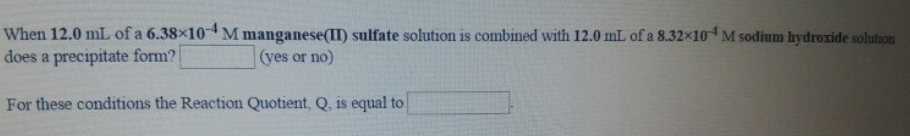# Problem: When 12.0 mL of a 6.38 x 10^-4 M manganese (II) sulfate solution is combined with 12.0mL of a 8.32 x 10^-4 M sodium hydroxide solution. For these conditions, the Reaction Quotient, Q, is equal to.

###### FREE Expert Solution
86% (33 ratings)###### Problem Details

When 12.0 mL of a 6.38 x 10^-4 M manganese (II) sulfate solution is combined with 12.0mL of a 8.32 x 10^-4 M sodium hydroxide solution. For these conditions, the Reaction Quotient, Q, is equal to.